# Machine Learning Lecture 11 Analytical Learning ExplanationBased Learning

• Slides: 11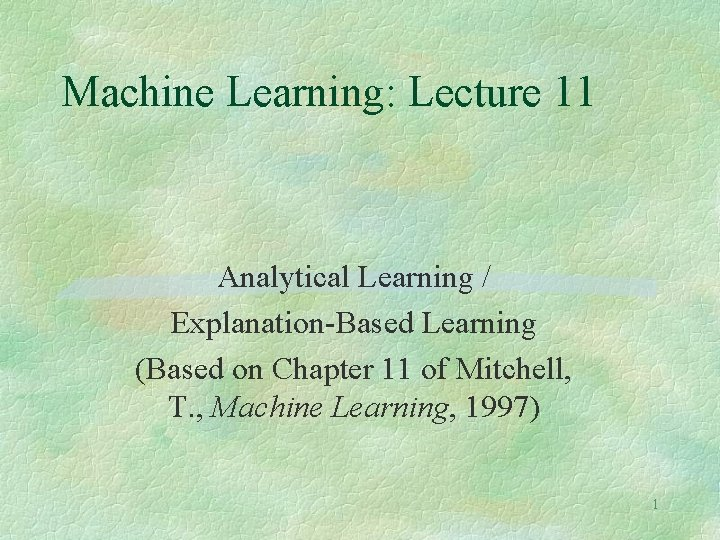Machine Learning: Lecture 11 Analytical Learning / Explanation-Based Learning (Based on Chapter 11 of Mitchell, T. , Machine Learning, 1997) 1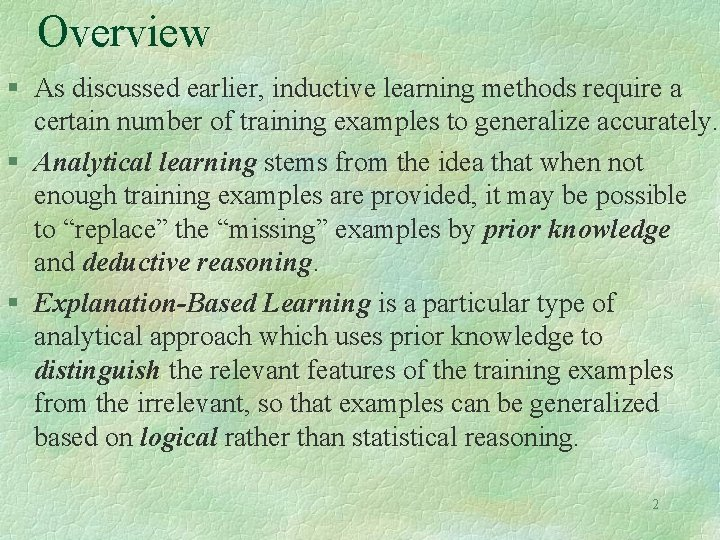Overview § As discussed earlier, inductive learning methods require a certain number of training examples to generalize accurately. § Analytical learning stems from the idea that when not enough training examples are provided, it may be possible to “replace” the “missing” examples by prior knowledge and deductive reasoning. § Explanation-Based Learning is a particular type of analytical approach which uses prior knowledge to distinguish the relevant features of the training examples from the irrelevant, so that examples can be generalized based on logical rather than statistical reasoning. 2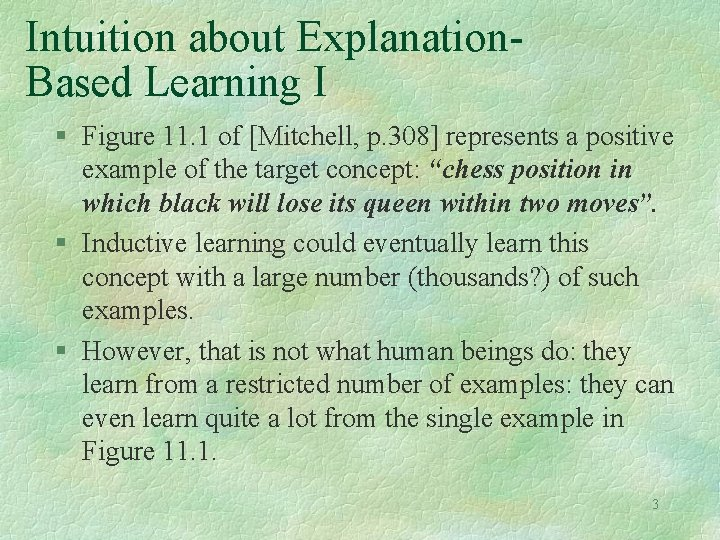Intuition about Explanation. Based Learning I § Figure 11. 1 of [Mitchell, p. 308] represents a positive example of the target concept: “chess position in which black will lose its queen within two moves”. § Inductive learning could eventually learn this concept with a large number (thousands? ) of such examples. § However, that is not what human beings do: they learn from a restricted number of examples: they can even learn quite a lot from the single example in Figure 11. 1. 3Intuition about Explanation-Based Learning II § From the single board on Figure 11. 1, humans can suggest the general hypothesis: “board positions in which the black king and queen are simultaneously attacked”. They would not even consider the (equally consistent) hypothesis “board positions in which four white pawns are still in their original position”! § They do so, because they rely heavily on explaining or analyzing the example in terms of their prior knowledge about the legal moves of chess. § Explanation-Based-Learning attempts to learn in the same fashion. 4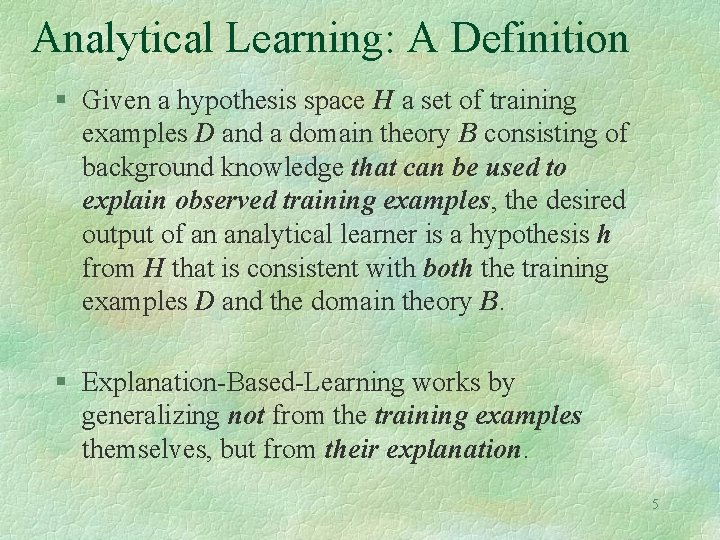Analytical Learning: A Definition § Given a hypothesis space H a set of training examples D and a domain theory B consisting of background knowledge that can be used to explain observed training examples, the desired output of an analytical learner is a hypothesis h from H that is consistent with both the training examples D and the domain theory B. § Explanation-Based-Learning works by generalizing not from the training examples themselves, but from their explanation. 5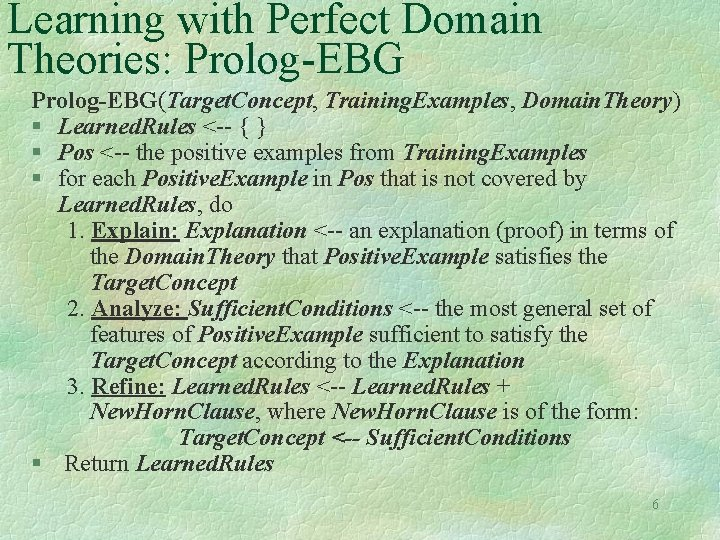Learning with Perfect Domain Theories: Prolog-EBG(Target. Concept, Training. Examples, Domain. Theory) § Learned. Rules <-- { } § Pos <-- the positive examples from Training. Examples § for each Positive. Example in Pos that is not covered by Learned. Rules, do 1. Explain: Explanation <-- an explanation (proof) in terms of the Domain. Theory that Positive. Example satisfies the Target. Concept 2. Analyze: Sufficient. Conditions <-- the most general set of features of Positive. Example sufficient to satisfy the Target. Concept according to the Explanation 3. Refine: Learned. Rules <-- Learned. Rules + New. Horn. Clause, where New. Horn. Clause is of the form: Target. Concept <-- Sufficient. Conditions § Return Learned. Rules 6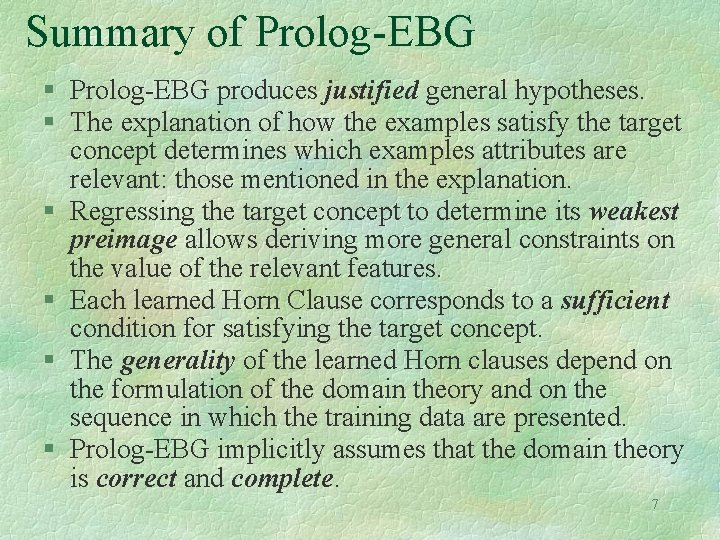Summary of Prolog-EBG § Prolog-EBG produces justified general hypotheses. § The explanation of how the examples satisfy the target concept determines which examples attributes are relevant: those mentioned in the explanation. § Regressing the target concept to determine its weakest preimage allows deriving more general constraints on the value of the relevant features. § Each learned Horn Clause corresponds to a sufficient condition for satisfying the target concept. § The generality of the learned Horn clauses depend on the formulation of the domain theory and on the sequence in which the training data are presented. § Prolog-EBG implicitly assumes that the domain theory is correct and complete. 7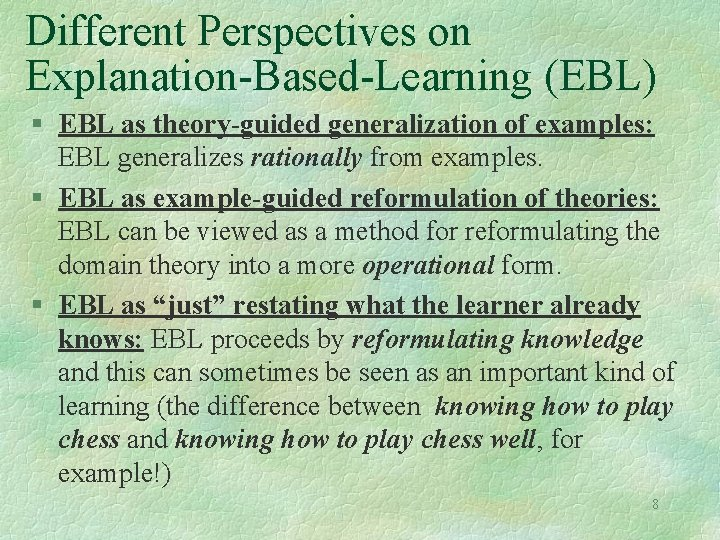Different Perspectives on Explanation-Based-Learning (EBL) § EBL as theory-guided generalization of examples: EBL generalizes rationally from examples. § EBL as example-guided reformulation of theories: EBL can be viewed as a method for reformulating the domain theory into a more operational form. § EBL as “just” restating what the learner already knows: EBL proceeds by reformulating knowledge and this can sometimes be seen as an important kind of learning (the difference between knowing how to play chess and knowing how to play chess well, for example!) 8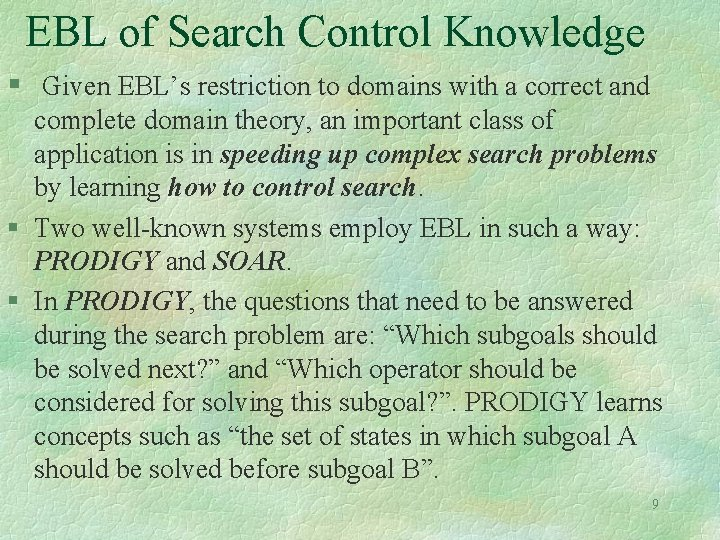EBL of Search Control Knowledge § Given EBL’s restriction to domains with a correct and complete domain theory, an important class of application is in speeding up complex search problems by learning how to control search. § Two well-known systems employ EBL in such a way: PRODIGY and SOAR. § In PRODIGY, the questions that need to be answered during the search problem are: “Which subgoals should be solved next? ” and “Which operator should be considered for solving this subgoal? ”. PRODIGY learns concepts such as “the set of states in which subgoal A should be solved before subgoal B”. 9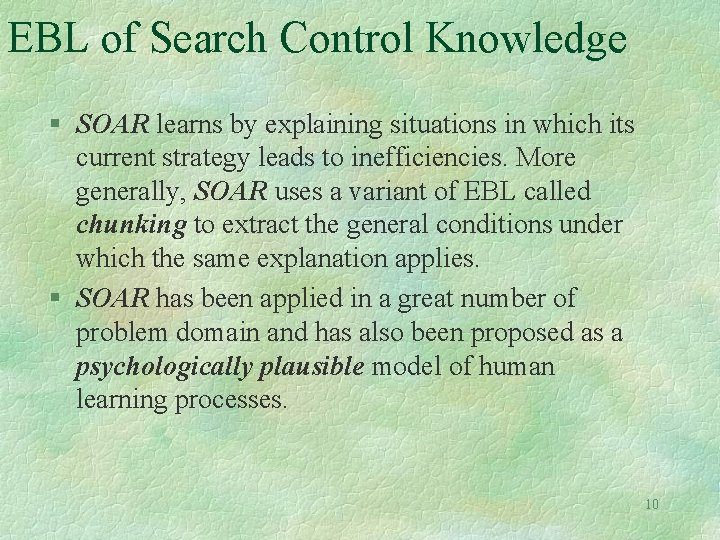EBL of Search Control Knowledge § SOAR learns by explaining situations in which its current strategy leads to inefficiencies. More generally, SOAR uses a variant of EBL called chunking to extract the general conditions under which the same explanation applies. § SOAR has been applied in a great number of problem domain and has also been proposed as a psychologically plausible model of human learning processes. 10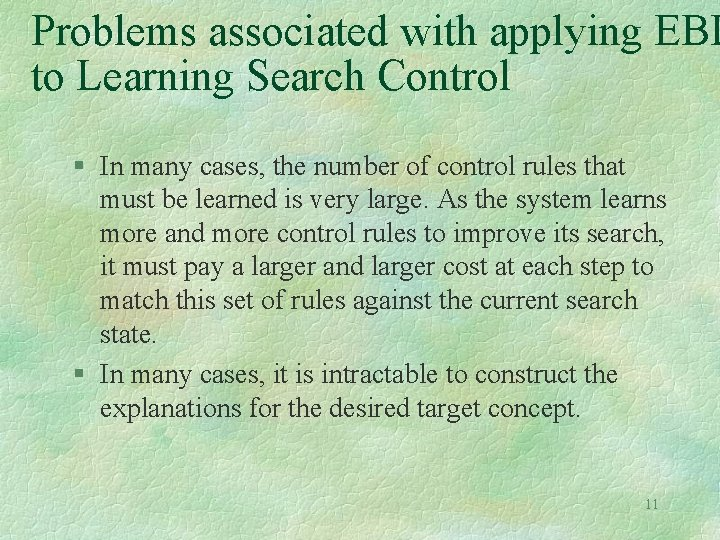Problems associated with applying EBL to Learning Search Control § In many cases, the number of control rules that must be learned is very large. As the system learns more and more control rules to improve its search, it must pay a larger and larger cost at each step to match this set of rules against the current search state. § In many cases, it is intractable to construct the explanations for the desired target concept. 11